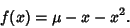## Flip Bifurcation

Let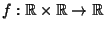be a one-parameter family of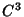maps satisfying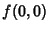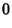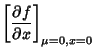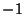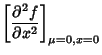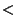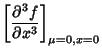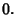Then there are intervals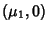,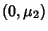, and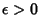such that
1. If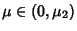, then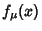has one unstable fixed point and one stable orbit of period two for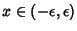, and

2. If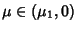, thenhas a single stable fixed point for.
This type of Bifurcation is known as a flip bifurcation. An example of an equation displaying a flip bifurcation is